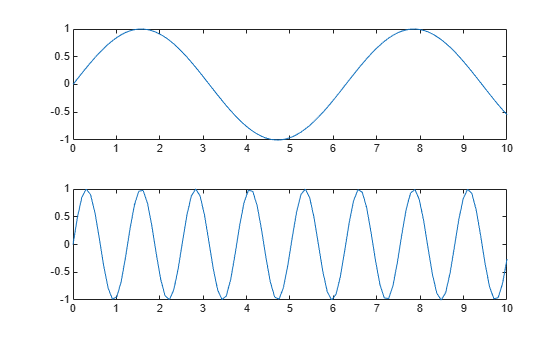# mlreportgen.finder.AxesFinder class

Package: mlreportgen.finder

Find axes in MATLAB figure window

## Description

Use an object of the `mlreportgen.finder.AxesFinder` class to find all axes in a MATLAB® figure window.

The `mlreportgen.finder.AxesFinder` class is a `handle` class.

### Class Attributes

 `HandleCompatible` `true`

For information on class attributes, see Class Attributes.

## Creation

### Description

example

````finder = mlreportgen.finder.AxesFinder(container)` creates an `mlreportgen.finder.AxesFinder` object and sets the Container property to a figure handle or path to a figure handle.```
````finder = mlreportgen.finder.AxesFinder(Name,Value)` sets properties by using name-value arguments. Specify multiple name-value arguments in any order.```

## Properties

expand all

Figure container, specified as a:

• Character vector or string scalar that contains the path to a valid figure file

• Valid figure handle

Properties of the axes to find, specified as a cell array of name-value arguments. The finder returns only axes that have the specified properties with the specified values.

expand all

## Examples

collapse all

This example shows how to use an `mlreportgen.finder.AxesFinder` object to find and include the axes in a given figure window.

Create a report.

```import mlreportgen.report.* import mlreportgen.finder.* rpt = Report("AxesFinder Example","pdf");```

Create a figure handle and plots to include in the report.

```f = figure; axes1 = subplot(2,1,1); x = linspace(0,10); y1 = sin(x); plot(x,y1); axes2 = subplot(2,1,2); y2 = sin(5*x); plot(x,y2);```Use the `find` method from `mlreportgen.finder.AxesFinder` to search for all axes currently open in the given figure window.

```customFinder = mlreportgen.finder.AxesFinder(f); results = customFinder.find;```

`append(rpt, results);````close(rpt); rptview(rpt);```